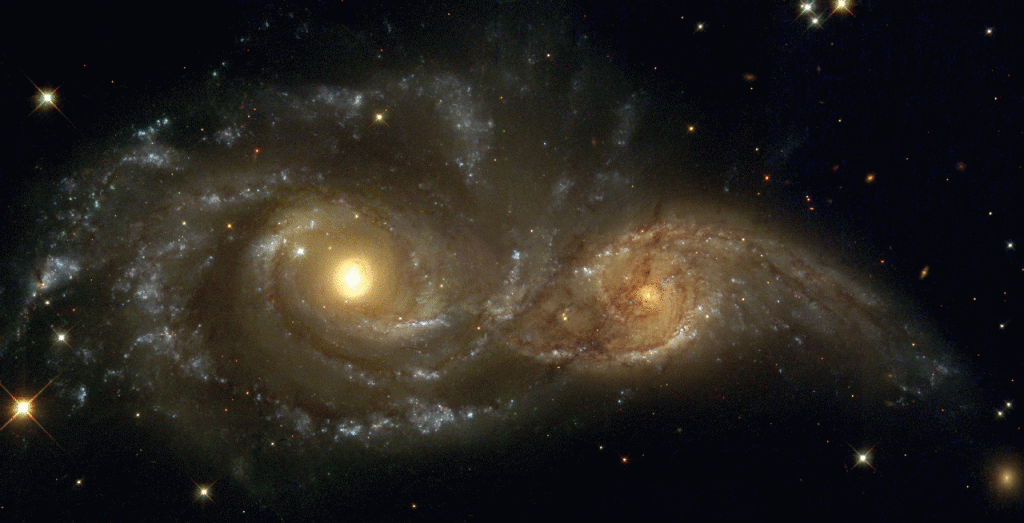# Something and Nothing

We should like to suggest a number of simple ideas relating to the origins of the physical Universe. These ideas are based as the title suggests on Something and Nothing.

Cosmological theory proposes a beginning to the universe which started out as a singularity about 13.8 billion years ago. This singularity contained all the energy of the galaxies, stars, planets and both interstellar and intergalactic matter as well as all the electromagnetic energy. We now (due to recent theories) have to add dark energy and dark matter.

This clearly was Something in the sense that it was a quantity of Energy. We suggest (see article on Dimensions) that this Energy manifested from other, higher, dimensions. But in this manifestation the Something paradoxically embodied, simultaneously, Nothing. In fact many Nothings:

1) Matter and antimatter; when they meet they annihilate each other and revert back to Electromagnetic energy.

2) Charge, equal amounts of positive and negative; when they meet the result is zero (perfect neutrality).

3) Linear momentum; as momentum is a vector (and has both magnitude and direction) when we sum all the momenta in the universe we arrive at exactly zero. The sum total of the momenta in any given direction will cancel the momenta in the opposite direction. This makes perfect sense in an expanding universe and suggests that there is no favoured direction.

4) Angular momentum; similar to linear momentum but in a rotational sense. In a singularity, having by definition no size, there cannot be any rotational characteristics. So as the universe expanded, if no Energy was added, then the sum total of the angular momenta must remain zero at all times. One problem does arise if we suggest an influx of new matter/energy i.e. dark matter and dark energy. However, as long as it also comes from a series of singularities then the total would still be absolutely Nothing.

This last conclusion leads to an explanation of one of the problems that lies at the heart of modern quantum theory, that of entanglement. The problem is this: if two particles (say electrons) are created simultaneously due to the collision of two gamma rays then they will occur as a standard electron and its anti-particle a positron. The positron has identical mass to the electron and the same charge but of opposite sign. The other quantity that is mirrored is that of spin; one electron will have a clockwise spin the other anticlockwise. Hence the sum total of their angular momenta is zero. This is in agreement with statement (4). If new particles have been created they cannot be seen to violate the law of “conservation of angular momentum”, meaning the total momentum before an event must be equal to the total after.

The problem known as entanglement arises if one of the electron was to undergo a collision and as a result reverse its direction of spin. It is a requirement of modern quantum theory that simultaneously the other electron, irrespective of where it is in the universe, will also change the direction of its spin. The difficulty here is that the physical universe seems to have a speed limit known as the speed of light. This limits all communications to occur over a period of time and prohibits the idea of such things occurring instantly.

The solution lies in the principle as stated above; the total angular momentum has to be maintained at zero so if one electron in the universe “flips” then another has to simultaneously “flip” the other way to compensate and uphold the Nothing principle. It is the mechanism behind this necessary adjustment that is the crux of the problem.

We suggest that when the two particles were created they were inextricably connected but via a higher dimension. This connection continues even after they separate. No matter what time we observe them in the future this entanglement still exists. Therefore when one is forced to change its direction of spin the other will do likewise and at exactly the same time! By analogy, imagine a seesaw: when we sit on one end it moves downwards and the other end simultaneously moves upwards. The reason being they are rigidly connected to each other.By working with data available for a number of nearby galaxies it became apparent that although their general motion was one of expansion and hence separation there is a case for suggesting rotation as well. This seems to occur with pairs and in some cases pairs of pairs of neighbouring galaxies. We discovered groups exhibiting clockwise angular momentum and others having exactly the same value but in an anticlockwise direction (the periods of these rotations were of the order of many times the age of the universe). This amazingly points towards “entanglement” between groups of galaxies not just something that exists between pairs of electrons. In conclusion we would suggest that “entanglement” may be a common occurrence and is found at both the microscopic and macroscopic levels.

So many of the problems of quantum physics, at one end of the scale, and cosmology at the other end can be solved in a very simple manner if we invoke the possibility of a multi-dimensional universe. This in turn gives rise to the idea that within a higher dimension all things in our 3 dimensional universe may be “entangled”.

“When we really begin to understand the universe I believe the answer will be so simple
that we will wonder why it took so long!”